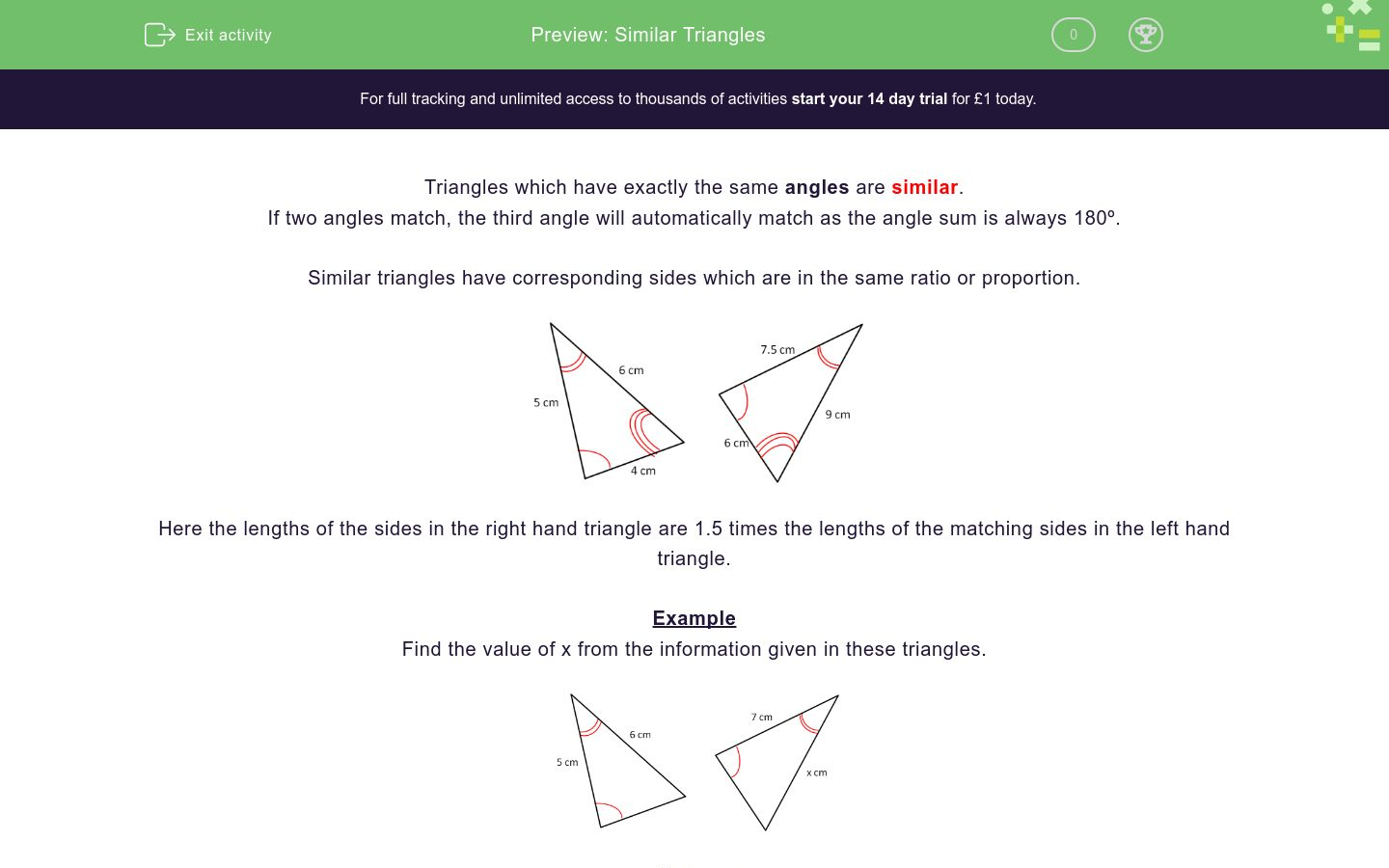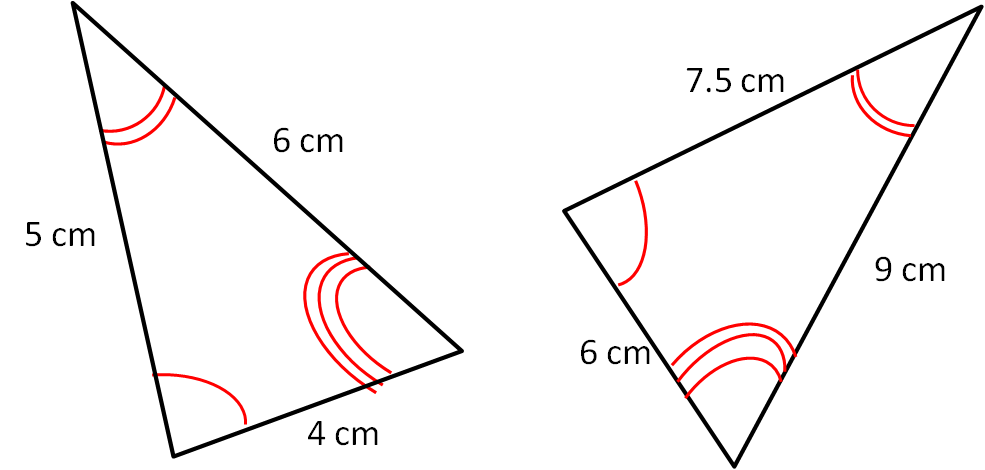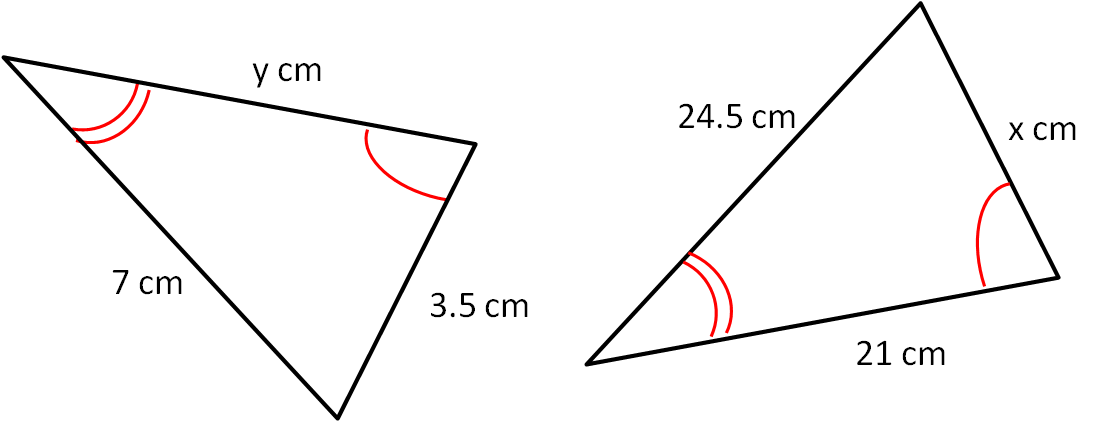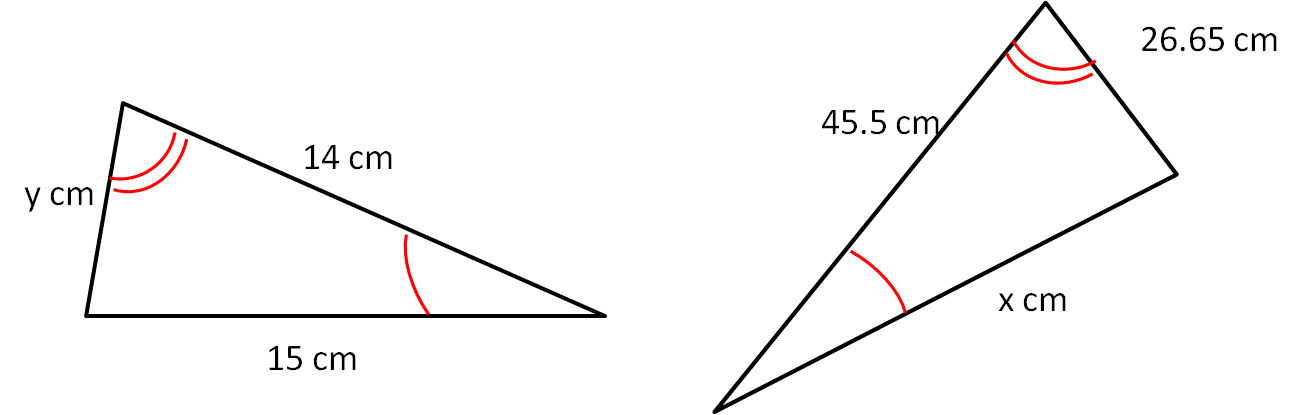# Similar Triangles

In this worksheet, students revise similar triangles and use proportions to work out the lengths of sides.Key stage:  KS 4

Curriculum topic:  Geometry and Measures

Difficulty level:### QUESTION 1 of 10

Triangles which have exactly the same angles are similar.

If two angles match, the third angle will automatically match as the angle sum is always 180º.

Similar triangles have corresponding sides which are in the same ratio or proportion.Here the lengths of the sides in the right hand triangle are 1.5 times the lengths of the matching sides in the left hand triangle.

Example

Find the value of x from the information given in these triangles.The triangles are similar because two angles match, and so will the third too.

Comparing corresponding sides, we get that the scale factor is 7 ÷ 5 = 1.4

x = 1.4 × 6 = 8.4

Find the value of x from the information given in these triangles.(not drawn to scale)

Find the value of y from the information given in these triangles.(not drawn to scale)

Find the value of x from the information given in these triangles.(not drawn to scale)

Find the value of y from the information given in these triangles.(not drawn to scale)

Find the value of x from the information given in these triangles.(not drawn to scale)

Find the value of y from the information given in these triangles.(not drawn to scale)

Find the value of x from the information given in these triangles.(not drawn to scale)

Find the value of y from the information given in these triangles.(not drawn to scale)

Find the value of x from the information given in these triangles.(not drawn to scale)

Find the value of y from the information given in these triangles.(not drawn to scale)

• Question 1

Find the value of x from the information given in these triangles.(not drawn to scale)

9.8
EDDIE SAYS
Scale factor = 7 ÷ 5 = 1.4
x = 1.4 × 7 = 9.8
• Question 2

Find the value of y from the information given in these triangles.(not drawn to scale)

4
EDDIE SAYS
Scale factor = 7 ÷ 5 = 1.4
y = 5.6 ÷ 1.4 = 4
• Question 3

Find the value of x from the information given in these triangles.(not drawn to scale)

5.6
EDDIE SAYS
Scale factor = 8 ÷ 5 = 1.6
x = 3.5 × 1.6 = 5.6
• Question 4

Find the value of y from the information given in these triangles.(not drawn to scale)

4.375
EDDIE SAYS
Scale factor = 8 ÷ 5 = 1.6
y = 7 ÷ 1.6 = 4.375
• Question 5

Find the value of x from the information given in these triangles.(not drawn to scale)

12.25
EDDIE SAYS
Scale factor = 24.5 ÷ 7 = 3.5
x = 3.5 × 3.5 = 12.25
• Question 6

Find the value of y from the information given in these triangles.(not drawn to scale)

6
EDDIE SAYS
Scale factor = 24.5 ÷ 7 = 3.5
y = 21 ÷ 3.5 = 6
• Question 7

Find the value of x from the information given in these triangles.(not drawn to scale)

63
EDDIE SAYS
Scale factor = 71.4 ÷ 17 = 4.2
x = 15 × 4.2 = 63
• Question 8

Find the value of y from the information given in these triangles.(not drawn to scale)

8
EDDIE SAYS
Scale factor = 71.4 ÷ 17 = 4.2
y = 33.6 ÷ 4.2 = 8
• Question 9

Find the value of x from the information given in these triangles.(not drawn to scale)

48.75
EDDIE SAYS
Scale factor = 45.5 ÷ 14 = 3.25
x = 15 × 3.25 = 48.75
• Question 10

Find the value of y from the information given in these triangles.(not drawn to scale)

8.2
EDDIE SAYS
Scale factor = 45.5 ÷ 14 = 3.25
y = 33.6 ÷ 3.25 = 8.2
---- OR ----

Sign up for a £1 trial so you can track and measure your child's progress on this activity.

### What is EdPlace?

We're your National Curriculum aligned online education content provider helping each child succeed in English, maths and science from year 1 to GCSE. With an EdPlace account you’ll be able to track and measure progress, helping each child achieve their best. We build confidence and attainment by personalising each child’s learning at a level that suits them.

Start your £1 trial